# Partial correlation coefficient

(diff) ← Older revision | Latest revision (diff) | Newer revision → (diff)

A measure of the linear dependence of a pair of random variables from a collection of random variables in the case where the influence of the remaining variables is eliminated. More precisely, suppose that the random variableshave a joint distribution in, and let,be the best linear approximations to the variablesand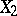based on the variables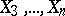. Then the partial correlation coefficient between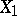and, denoted by, is defined as the ordinary correlation coefficient between the random variables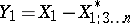and:It follows from the definition that. The partial correlation coefficient can be expressed in terms of the entries of the correlation matrix. Let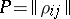, where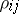is the correlation coefficient betweenand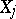, and let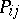be the cofactor of the elementin the determinant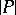; then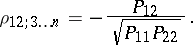For example, for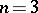,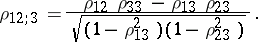The partial correlation coefficient of any two variables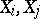fromis defined analogously. In general, the partial correlation coefficient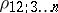is different from the (ordinary) correlation coefficient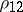ofand. The difference betweenandindicates whetherandare dependent, or whether the dependence between them is a consequence of the dependence of each of them on. If the variablesare pairwise uncorrelated, then all partial correlation coefficients are zero.

The empirical analogue of the partial correlation coefficient, the empirical partial correlation coefficient or sample partial correlation coefficient is the statistic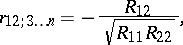where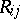is the cofactor in the determinant of the matrix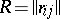of the empirical correlation coefficients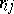. If the results of the observations are independent and multivariate normally distributed, and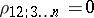, thenis distributed according to the probability density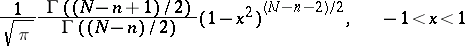(is the sample size). To test hypotheses about partial correlation coefficients, one uses the fact that the statistichas, under the stated conditions, a Student distribution with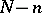degrees of freedom.